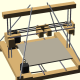# Unser aktuelles ABS Slic3r Profil

Es ist ein Slic3r Profil für ABS und den GRRF PRotos Drucker. Wir verwenden die Slic3r Version 0.9.3:

```# generated by Slic3r 0.9.3 on Wed Oct 31 17:14:28 2012 acceleration = 0 bed_size = 200,200 bed_temperature = 110 bridge_fan_speed = 100 bridge_flow_ratio = 1 bridge_speed = 70 brim_width = 0 complete_objects = 0 cooling = 1 disable_fan_first_layers = 1 duplicate = 1 duplicate_distance = 6 duplicate_grid = 1,1 end_gcode = M107\n; M104 S0 ; turn off extruder temperature\n; M140 S0 ; turn off plate\nM104 S255 ; turn off extruder temperature\nM140 S110 ; turn off plate\nG28 X0 ; home X axis\nG28 Y0 ; home Y axis\n;G1 Y+100\nM84 ; disable motors\n; M240 external_perimeter_speed = 100% extra_perimeters = 1 extruder_clearance_height = 20 extruder_clearance_radius = 20 extruder_offset = 0x0 extrusion_axis = E extrusion_multiplier = 3.9 extrusion_width = 0 fan_always_on = 1 fan_below_layer_time = 60 filament_diameter = 2.9 fill_angle = 90 fill_density = 0.6 fill_pattern = rectilinear first_layer_bed_temperature = 110 first_layer_extrusion_width = 0 first_layer_height = 85% first_layer_speed = 80% first_layer_temperature = 255 g0 = 0 gcode_arcs = 0 gcode_comments = gcode_flavor = reprap infill_acceleration = 50 infill_every_layers = 1 infill_extruder = 1 infill_extrusion_width = 0 infill_speed = 70 layer_gcode = ; M240 layer_height = 0.4 max_fan_speed = 100 min_fan_speed = 100 min_print_speed = 10 notes = nozzle_diameter = 0.45 only_retract_when_crossing_perimeters = 0 output_filename_format = [input_filename_base]_PRotos.gcode perimeter_acceleration = 25 perimeter_extruder = 1 perimeter_extrusion_width = 0 perimeter_speed = 40 perimeters = 2 post_process = print_center = 100,100 randomize_start = 1 retract_before_travel = 4 retract_length = 4 retract_length_toolchange = 3 retract_lift = 0.1 retract_restart_extra = 0 retract_restart_extra_toolchange = 0 retract_speed = 200 rotate = 0 scale = 1 skirt_distance = 5 skirt_height = 1 skirts = 3 slowdown_below_layer_time = 15 small_perimeter_speed = 40 solid_fill_pattern = rectilinear solid_infill_below_area = 70 solid_infill_every_layers = 0 solid_infill_speed = 70 solid_layers = 2 start_gcode = ;PRotos Profile\nG28 ; home all axis\n;M301 P13.82 I0.84 D56.60 ;2PrintBeta Heater\nM301 P26.89 I1.42 D127.33 ;GRRF Heater\n;M301 P50.76 I4.08 D158.02 support_material = support_material_angle = 0 support_material_extruder = 1 support_material_extrusion_width = 0 support_material_pattern = honeycomb support_material_spacing = 2.5 support_material_threshold = 45 temperature = 255 threads = 10 top_solid_infill_speed = 50 travel_speed = 150 use_relative_e_distances = 0 z_offset = 0 ```

Unser Profil ist eine angepasste Kopie von Thomas’s Slic3r Profil.

Veröffentlicht von in 3D Drucker

### E-Mail Benarichtigung bei neuen Artikeln

Bitte hier E-Mail-Adresse eingeben wenn Sie benachrichtigt werden möchten bei neuen Artikeln.

Schließe dich 790 anderen Followern anxandr bei Schritt Motor reparieren Links zum Wochenende… bei CNC QA auf dem Barcamp Mü…noktok bei Prusa i3 MK2S — Nach 6 Jahren…HML bei Prusa i3 MK2S — Nach 6 Jahren…marhilbru bei Prusa i3 MK2S — Nach 6 Jahren…Gentleman Nerd bei Prusa i3 MK2S — Nach 6 Jahren…Christian S bei Prusa i3 MK2S — Nach 6 Jahren…brumi28 bei CNC QA auf dem Barcamp Mü… Links zum Wochenende… bei CNC QA auf dem Barcamp Mü…CNC QA auf dem Barca… bei Elektronik-Hardware der CNC-Fr…#BlogBlick No. 7 | P… bei CNC  1#BlogBlick No. 7 | P… bei CNC Projekt 2016deloarts bei CNC Projekt 2016nathanbruec bei CNC Projekt 2016deloarts bei CNC Projekt 2016